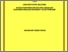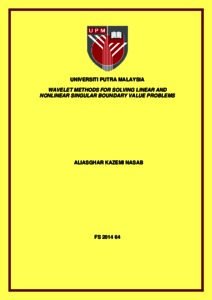# Wavelet methods for solving linear and nonlinear singular boundary value problems

## Citation

Nasab, Aliasghar Kazemi (2014) Wavelet methods for solving linear and nonlinear singular boundary value problems. Doctoral thesis, Universiti Putra Malaysia.

## Abstract

In this thesis, wavelet analysis method is proposed for solving singular boundary value problems. Operational matrix of differentiation is introduced. Furthermore, product operational matrix is also presented. Many different examples are solved using Chebyshev wavelet analysis method to confirm the accuracy and the efficiency of wavelet analysis method. An efficient and accurate method based on hybrid of Chebyshev wavelets and finite difference methods is introduced for solving linear and nonlinear singular ordinary differential equations such as Lane-Emden equations, boundary value problems of fractional order and singular and nonsingular systems of boundary and initial value problems. High-order multi-point boundary value problems are also solved. The useful properties of Chebyshev wavelets and finite difference method make it a computationally efficient method to approximate the solution of nonlinear equations in a semi-infinite interval. The given problem is converted into a system of algebraic equations using collocation points. The main advantage of this method is the ability to represent smooth and especially piecewise smooth functions properly. It is also clarified that increasing the number of subintervals or the degree of the Chebyshev polynomials in a proper way leads to improvement of the accuracy. Moreover, this method is applicable for solving problems on large interval. Several examples will be provided to demonstrate the powerfulness of the proposed method. A comparison is made among this method, some other well-known approaches and exact solution which confirms that the introduced method are more accurate and efficient. For future studies, some problems are proposed at the end of this thesis.Preview
Text
FS 2014 64 IR.pdfView Item